# Real estate

The residential house has three entrances numbered by odd numbers arithmetic progression. The sum of the two numbers on the corner entrances is 50. Calculate the highest of these three numbers.

m =  27

### Step-by-step explanation:

m+(m-4)=50

2m = 54

m = 27

Our simple equation calculator calculates it.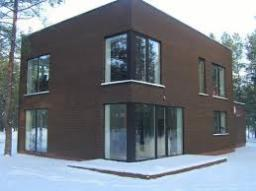Did you find an error or inaccuracy? Feel free to write us. Thank you!Tips to related online calculators
Do you solve Diofant problems and looking for a calculator of Diofant integer equations?
Do you have a linear equation or system of equations and looking for its solution? Or do you have a quadratic equation?

## Related math problems and questions:

• House numbering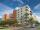The residential house has three entrances numbered even numbers, successive immediately behind. The sum of the two numbers on the outside entrances is 68. Calculate the middle of these three numbers.
• Odd numbersThe sum of four consecutive odd numbers is 1048. Find those numbers ...
• Digit sum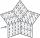How many are three-digit numbers that have a digit sum of 6?
• Sum of odd numbersFind the sum of all odd integers from 13 to 781.
• Sum of the seventeen numbersThe sum of the 17 different natural numbers is 154. Determine the sum of the two largest ones.
• Z9-I-4Kate thought a five-digit integer. She wrote the sum of this number and its half at the first line to the workbook. On the second line wrote a total of this number and its one fifth. On the third row, she wrote a sum of this number and its one nines. Fina
• AM of three numbersThe number 2010 can be written as the sum of 3 consecutive natural numbers. Determine the arithmetic mean of these numbers.
• The sumThe sum of five consecutive odd numbers is 75. Find out the sum of the second and fourth of them.
• 7 digit numberIf 3c54d10 is divisible by 330, what is the sum of c and d?
• Diofant equation250x + 120y = 5640
• The HotelThe Holiday Hotel has the same number of rooms on each floor. Rooms are numbered with natural numerals sequentially from the first floor, no number is omitted, and each room has a different number. Three tourists arrived at the hotel. The first one was in
• Even / odd numbers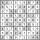a / Using variable n write two consecutive odd numbers. b / the sum of three consecutive odd numbers is 333. What are this numbers?
• DivisibilityDetermine the smallest integer which divided 11 gives remainder 4 when divided 15 gives remainder 10 and when divided by 19 gives remainder 16.
• RemainderA is an arbitrary integer that gives remainder 1 in the division with 6. B is an arbitrary integer that gives remainder 2 the division by. What makes remainder in division by 3 product of numbers A x B ?
• Sum of sevenThe sum of seven consecutive odd natural numbers is 119. Determine the smallest of them.
• Pine's forest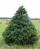There were so many pines in the forest that they were sequentially numbered 1, 2, 3,..., would use three times more digits than the pine trees alone. How many pine trees were there in the forest?
• Insurance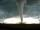The house owner is insured against natural disasters and pays 0.04% annually of the value of house 53 Eur. Calculate the value of the house. Calculate the probability of disaster if you know that 41% of the insurance is to pay damages.